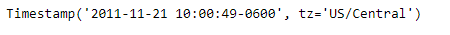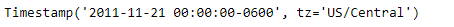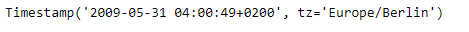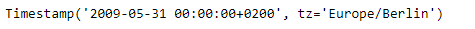Open in App
Not now

# Python | Pandas Timestamp.normalize

• Last Updated : 14 Jan, 2019

Python is a great language for doing data analysis, primarily because of the fantastic ecosystem of data-centric python packages. Pandas is one of those packages and makes importing and analyzing data much easier.

Pandas` Timestamp.normalize()` function is used to normalize Timestamp to midnight. The function perform this operation while preserving tz information.

Syntax :Timestamp.normalize()

Parameters : None

Return : Timestamp

Example #1: Use `Timestamp.normalize()` function to normalize the Timestamp to midnight value.

 `# importing pandas as pd``import` `pandas as pd`` ` `# Create the Timestamp object``ts ``=` `pd.Timestamp(year ``=` `2011``,  month ``=` `11``, day ``=` `21``,``                  ``hour ``=` `10``, second ``=` `49``, tz ``=` `'US/Central'``)`` ` `# Print the Timestamp object``print``(ts)`

Output :Now we will use the `Timestamp.normalize()` function to normalize the value in the given Timestamp to midnight value.

 `# normalize to midnight value``ts.isoweekday()`

Output :As we can see in the output, the `Timestamp.normalize()` function has normalized the given Timestamp to midnight.

Example #2: Use `Timestamp.normalize()` function to normalize the Timestamp to midnight value.

 `# importing pandas as pd``import` `pandas as pd`` ` `# Create the Timestamp object``ts ``=` `pd.Timestamp(year ``=` `2009``, month ``=` `5``, day ``=` `31``,``                  ``hour ``=` `4``, second ``=` `49``, tz ``=` `'Europe/Berlin'``)`` ` `# Print the Timestamp object``print``(ts)`

Output :Now we will use the `Timestamp.normalize()` function to normalize the value in the given Timestamp to midnight value.

 `# normalize to midnight value``ts.isoweekday()`

Output :As we can see in the output, the `Timestamp.normalize()` function has normalized the given Timestamp to midnight.

My Personal Notes arrow_drop_up